Unlimited PowerPoint templates, graphics, videos & courses! Unlimited asset downloads! From $16.50/m Advertisement # How to Use Simple IF Statements in Excel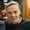Read Time: 9 mins Excel is one of the most powerful and easiest-to-use tools for working with data. A spreadsheet is really just a tool for organizing, calculating, and reviewing your data with. What happens when we need to build options into our spreadsheet? This is where if statements really come into play. Imagine that we have a spreadsheet with a list of scores from our school courses. I want to remind myself if I'm passing or failing a class with a simple "passing" or "failing" text. I can write an IF statement formula to look at the final grade, and then print "passing" or "failing" based on the score.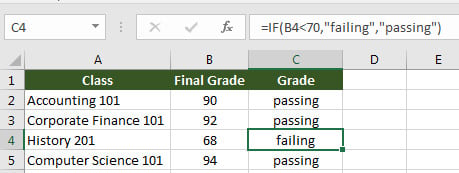This simple IF statement example looks at the score in column B and reminds me if I'm passing or failing a class based on the score. An Excel IF formula makes your spreadsheets much smarter. We can give a cell different choices for what to show based on a condition. An IF formula lets us build in logic to our spreadsheet. In this tutorial, I'll teach you how to use them. ## How to Use Excel IF Statements (Video Tutorial) In this screencast, you'll see how I use IF statements in Excel to make a spreadsheet smarter. Watch this video to walk through building IF statements, and then check out the tutorial below for written instructions. Before we move on with the tutorial, I'd recommend downloading the free example workbook I've created for this tutorial. ## How Does an Excel IF Statement Work? There are three key parts to a successful IF statement: 1. Something to Check - Basically, what should the IF statement check for? We could check to see if a cell equals a certain number, or to see if it contains a certain string of text, for example. 2. What to Show If True - If the statement contains what we're checking for, what should the cell show? 3. What to Show If False - If the statement doesn't contain what we're looking for, what should the fallback be? An Excel IF statement begins with =IF( .The official Excel documentation shows the structure of an Excel IF statement: =IF(logical_test,[value_if_true],[value_if_false]) In plain English, here's how I think of using an IF Statement: =IF(what to check For, what to show if true, what to show if false) Simple enough? If this still seems a bit vague, let's move onto our very first example of how to use an IF statement, to see how it works. ## Writing Your First IF Statement For this example, use the tab titled "Check for Blank" in the example workbook. We'll start off with the simplest possible IF statement example. In this case, we're just going to check to see if a cell is blank and print a message. Let's say that we're creating a sign up list for our office holiday party. We need to write a formula to determine who to remind to sign up for a dish. At the beginning, our data is a simple list of names and what they've signed up to bring: Even though I can see that some cells are blank, let's write an IF formula to print out who we need to remind in column D. My IF formula should check if the cells in column C are blank, and print a reminder to the person if they've not signed up yet. Here's what I wrote: =IF(C2="","remind them!","they've already signed up.") Let's dissect this formula in four parts: 1. Every IF statement opens with =IF( 2. Next, I'm going to check if the values in column C are empty, so I wrote C2="",Writing two quotation marks with no text between them will check to see if a cell is empty. The comma at the end helps us move onto the next part of the formula. 3. The next part of the formula is what will show anytime the formula is true. Since we're checking to see if the cell is blank, we're going to print "remind them!" when it is blank. 4. If C2 does contain some text, we'll print that the person has already signed up. And finally, here's the results when we finish the formula and pull it down: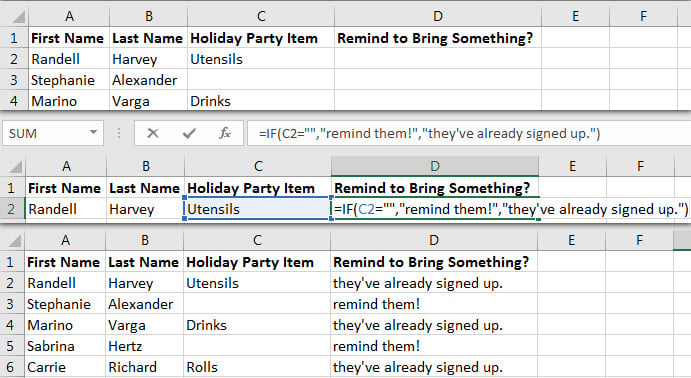After writing the IF statement and pulling it down, each cell in column D outputs the correct value. Perfect! Notice that when the adjacent cell in column C is blank, Excel prints that we need to remind that person to sign up. We could filter for Column D now and then send them a reminder via email. This is a simple example for how we can use if statement formulas in Excel to give our spreadsheets some logic. Let's look at some others. ## Testing for Values Use the Test Values tab in the example workbook for this exercise. So far, we used an IF statement to see if a cell contained no text. Now, let's use an IF statement to test for a numerical value. In the first example, we checked to see if the cells in column C contained a specific bit of text. Notice that we used the equals sign to see if a cell was equal to a blank. We can use any of the math operators to test for values, such as: • - check to see if a cell is equal to a specific value • <> - check to see if a cell is not equal to a specific value • - check to see if a cell is greater than the value in the formula • < - check to see if a cell is less than the value in the formula • >= - check to see if a cell is greater than or equal to the value in the formula • <= - check to see if a cell is less than or equal to the value in the formula Let's say that we need to take inventory of our warehouse. We sell our product in batches of 10, so every batch we count should have exactly that amount. Let's write a formula to check for it. In the data below, I've gone out and taken stock of products in my warehouse. I need to write a formula in column E to check and make sure that we have exactly 10 of each product. We should print a warning message if we don't have 10 of each item. In column E, here's the formula that I'll write: =IF(D4<>10,"needs attention","") Let's dissect the formula: • First, I use the <> operator to check to see if the value in cell D4 doesn't equal 10, which indicates a problem. • Next, I've got a message in quotations of "needs attention" which will show anytime the adjacent cell isn't 10. • Finally, I add a comma and two quotation marks with nothing between them so that if the count was 10, my formula simply prints nothing.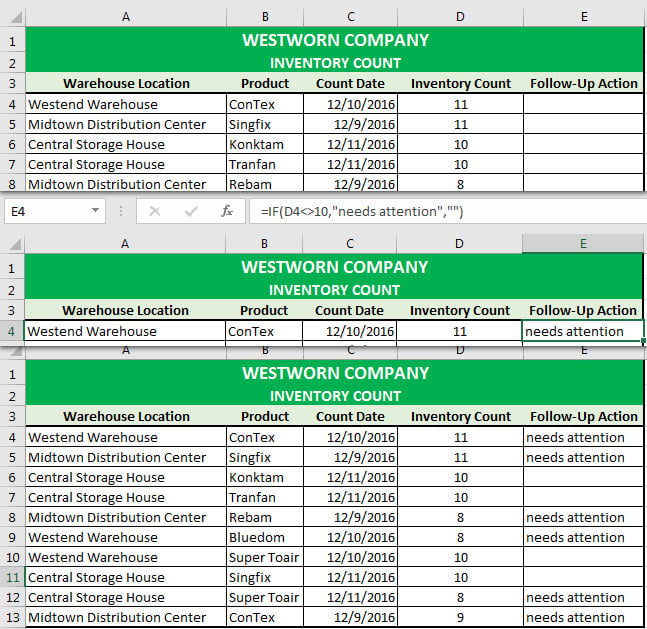Needs attention follow-up action result. In the screenshot above, you'll see how I used the formula to add warning messages for each of my inventory counts. If the value in column D isn't exactly 10, Excel will print "needs attention" in column E. ## How to Use IFERROR There's a special IF statement formula built into Excel for fixing errors in your formulas. The IFERROR formula is similar to an IF statement, but catches a wide array of errors and replaces them with more useful data. According to Microsoft's documentation, here are errors in your spreadsheet that IFERROR can catch and revise: • #N/A • #VALUE! • #REF! • #DIV/0! • #NUM! • #NAME? • #NULL! To use IFERROR, bracket an existing formula with an IFERROR formula, and then provide a fallback value. This is how I think of using IFERROR: =IFERROR([your existing formula],[what to replace the error with]) Let's walk through an extremely useful case for IFERROR. ### An IFERROR Example Use the IFERROR tab in the example workbook for this section. One place that you'll run into errors is with the VLOOKUP formula. If you're using a VLOOKUP to match values, there's a chance that your lookup table won't contain a match. When that happens, you're going to get an "N/A" error in your spreadsheets. In the spreadsheet below, I've got a list of freelancers working for me on a job. I've got their company listed, and want to know what each person is doing. For most of the freelancers, I have a lookup table to the right that's pulling in their roles.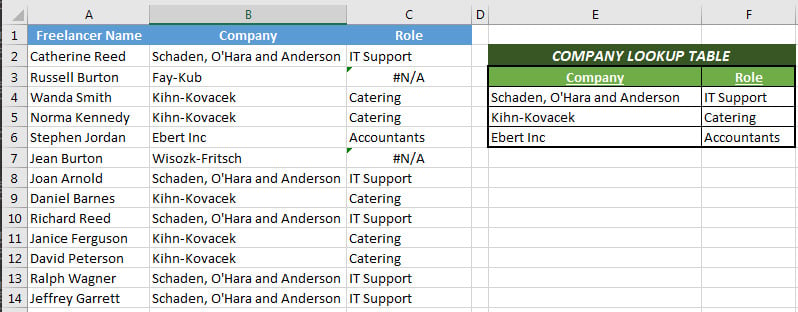This spreadsheet has some N/A errors because my lookup table is missing matches. I've already written a VLOOKUP to pull in the job role, which matches the companies in column B with my lookup table: =VLOOKUP(B2,$E$4:$F$6,2,FALSE) Here's the problem: several of the companies aren't in my lookup table, which is why we have these nasty N/A errors. Let's replace them with something more elegant. The IFERROR that we'll want to write will follow this structure: =IFERROR(the existing formula, what to replace an error with) In my case, I'm going to replace the errors with a reminder to contact my HR manager to check what the freelancer is working on. Here's my proposed formula: =IFERROR(VLOOKUP(B3,$E$4:$F\$6,2,FALSE),"Contact HR")

This formula looks complex, but just remember: all that we're doing is bracketing an existing VLOOKUP with a replacement value. We simply surround our current formula with an IFERROR and give Excel a fallback value.

In short, IFERROR is a special IF statement that Microsoft designed for our convenience. It automatically catches formula errors and outputs a replacement value.

## Recap and Keep Learning

IF statements are a method to add conditional logic to your spreadsheets. In this tutorial, you learned to use the formula and some possible uses for it in your spreadsheets.

No matter how long I use Excel, I keep finding out that I have more to learn. If you want to keep learning how spreadsheets can be used, here are some follow-up tutorials:

• The official Microsoft documentation is always my first stop when learning a new formula, and their page on IF statements is no slouch.
• If you're thinking about IF statements, you're probably on the advanced side of Excel users. A complementary skill is using VLOOKUP to match elements of lists.
• If you want to get advanced, try this Nested IF functions tutorial from Exceljet. Instead of a simple IF statement that has only two possible outcomes, nesting a combination of these formulas give you more options.

If you have an Excel IF statement issue, let me know in the comments. Or, if you have ideas on how to use these formulas to share with other readers, the comments are open.